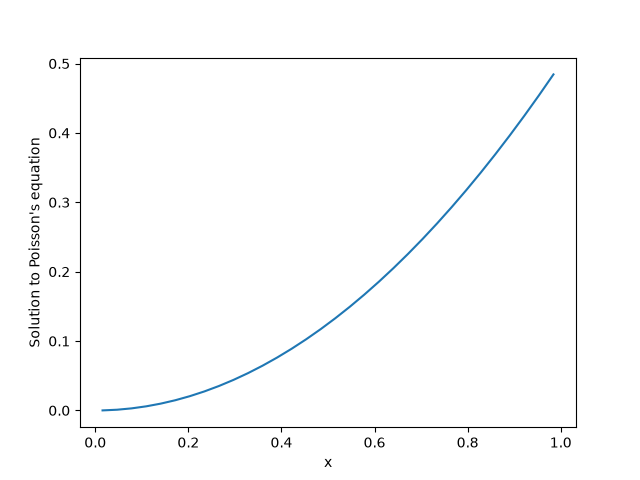# 2.4. Solving Poisson’s equation in 1d¶

This example shows how to solve a 1d Poisson equation with boundary conditions.```from pde import CartesianGrid, ScalarField, solve_poisson_equation

grid = CartesianGrid([[0, 1]], 32, periodic=False)
field = ScalarField(grid, 1)
result = solve_poisson_equation(field, bc=[{"value": 0}, {"derivative": 1}])

result.plot()
```

Total running time of the script: ( 0 minutes 0.082 seconds)

Gallery generated by Sphinx-Gallery